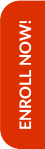Olympiads are the stepping stones to achieve better results in the competitive world that lies ahead in the life of the child. Math Olympiad examinations help students to improve their mathematical skills along with their analytical and problem solving abilities. Along with that CBSE syllabus also plays a crucial role in the same.

Hence, Olympiad Success Live has designed a long-term course for Mathematics preparation which includes both CBSE syllabus and Olympiad for class 6. This long-term course will help in building the foundation of the child. For this, we have done great efforts in finding the tutor for class 6 Math CBSE and Olympiad preparation with relevant background and experience.

If you are interested in purchasing this course, then please Enrol Now. You will be redirected to the batch detail page, wherein you can see all the details like batch start and demo dates, fess and the registration link related to Math CBSE and Olympiad for class 6 course.

Grade 6 CBSE Mathematics syllabus identifies age-appropriate content. During the learning of Mathematics from grade 6 to grade 8, a child:

• Moves from number sense to number patterns;
• Sees relationships between numbers and looks for patterns in relationships;
• Gains proficiency in using the newer language of Mathematics like variables, expressions, equations, identities, etc.
• Uses arithmetic and algebra to solve real-life problems and pose meaning problems;
• Discovers symmetries and acquire a sense of aesthetics by looking around regular shapes like triangles, circles, quadrilaterals, etc;
• Comprehends the idea of space as reason enclosed within boundaries of a shape;
• Relates numbers with shapes in terms of perimeter, area and volume and uses them to solve everyday life problems;
• Learns to provide reasoning and convincing arguments to justify her/his own conclusions particularly in Mathematics; and
• Collects, represents (graphically and in tables) and interprets data/information from her/his life experiences

The content of grade 6 CBSE Maths is consistent with the development of the student which includes:

• Numbers
• HCF and LCM
• Algebra
• Ratio and Proportion
• Geometry
• Mensuration
• Data Handling

Check the detailed syllabus below for CBSE preparation. For Olympiads preparation, you can check the syllabus here!

Keeping in mind the curricular expectations, Olympiad Success Live has designed a course for CBSE and Olympiads Maths preparation for class 6 students. And for this, we have the right set of tutors with relevant backgrounds and experience for class 6 Maths CBSE and Olympiads preparation.

If you are interested in enrolling in this grade 6 CBSE and Olympiads Math course, then you can pay the fees here. After payment, kindly WhatsApp us your name, class and subject at +91 95607 64447

##### Syllabus

Numbers

• Sense of numberness up to 5 digits in terms of its size of estimation
• Large numbers up to 8 digits
• Word problems on large number operations
• Brackets and other symbols like =, <, >
• Divisibility rules of 2, 3, 4, 5, 10
• Classification of numbers as even, old, prime, co- prime, etc.

HCF and LCM

• HCF and LCM
• Prime factorization
• Rules for whole numbers
• Properties of whole numbers like commutative, associative, distributive, additive identity, multiplicative identity, etc.
• Negative numbers.
• Ordering of integers, their representation on number line, addition and subtraction of integers, etc.
• Represent fractions and decimals pictorially and on number line
• Sum and difference of two fractions

Algebra

• Variables through patterns
• Quantities as variable and constant
• Algebra as generalisation of arithmetic

Ratio and Proportion

• Comparison of two quantities through ratio
• Meaning of proportion
• Relation of ratio and proportion to unitary method

Geometry

• Difference between different geometrical figures
• Open and closed figures
• Interior and exterior of closed figures
• Line, line segment, ray, curvilinear and linear boundaries
• Elements of angle like vertices, arms, interior and exterior
• Difference between different types of triangles and the basis on which they are classified
• Vertices, sides, angles, interior and exterior, altitude and median of a triangle
• Quadrilaterals as trapezium, parallelogram, rectangle, square, rhombus
• Circle and its components like centre, radius, diametre, arc, sector, chord, segment, semicircle, circumference, interior and exterior
• 3-D shapes and their elements
• 2-D symmetrical objects
• Reflection symmetry
• Angles of different measures
• Perpendicular line segments

Mensuration

• Perimeter and area of a shape

Data Handling

• Organizing data using tally marks
• Representing data through pictograph, bar graph

Online classes started for English, Mathematics, Science and Reasoning for classes (3rd to 10th)